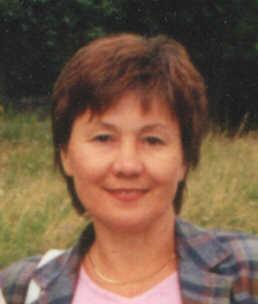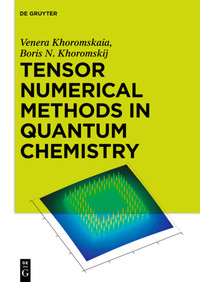#### Venera Khoromskaia, Dr. rer. nat.

Welcome to my homepage!Google Scholar profile

#### Talks at the conferences and seminars

```  Address:
Dr. Venera Khoromskaia
Max-Planck-Institute for Mathematics in the Sciences
Inselstr., 22-26, D-04103,
Leipzig, Germany

Tel.:  ++49 341-99 59 725
e-mail: vekh [at] mis.mpg.de

```
Some of the results of my work at the Max-Planck Institute for Mathematics in the Sciences:

Development of tensor numerical methods (since 2006)
Reduced higher order singular value decomposition (RHOSVD) as part of the canonical-to-Tucker (C2T) tensor transform (2006-2007)
Calculation of multi-dimensional (convolution) integrals in 1D complexity (2006-2010)
Tensor-based solver(s) for the Hartree-Fock equation (2009-2013).
Calculation of the 4D two-electron integrals (TEI) tensor in a form of low-rank Cholesky factorization by "1D density fitting" (2012).
Superfast tensor-based summation of long-range electrostatic potentials on finite 3D lattices (2013-2015)
Bethe-Salpeter excitation energies using low-rank tensor factorizations.
Fast computation of the density of states for optical spectra of molecules.
Range-separated tensor format for numerical modeling of multiparticle systems of general type. (2016-2017)
Tensor numerical modeling of 3D collective electrostatics in many-particle systems. (2013-2020)These and other interesting topics (with examples in MATLAB) are discussed in the recent book:

Venera Khoromskaia and Boris N. Khoromskij
Tensor Numerical Methods in Quantum Chemistry
De Gruyter, Berlin, 2018.

I received University Diploma in Physics in 1974, and next 21 years I have been working at the Joint Institute for Nuclear Research (JINR) in Dubna near Moscow, which at that time had close collaboration with CERN in Geneva. I took part in processing of large scale data from experiments on particles accelerators in high energy physics.

In 2006 I started working at the Max-Planck Institute for Mathematics in the Sciences on Tucker tensor decomposition for function related tensors (together with Boris Khoromskij). This application revealed a number of properties of the Tucker tensor format which were not known before. It showed that for multidimensional tensors obtained by discretization of some classes of multivariate functions on Cartesian grids, the error of the Tucker tensor approximation decays exponentially in the Tucker rank.

On the basis of this knowledge we developed the canonical-to-Tucker decomposition (C2T, 2007) and the reduced higher order singular value decomposition (RHOSVD), which promoted the tensor formats ``avoiding the curse of dimensionality'' (since RHOSVD does not need the construction of a full size tensor). In 2006-2008 we have shown that when using the rank-structured tensor representation for functions and operators, calculation of the 3D convolution integrals with the Newton kernel is reduced to a sequence of one-dimensional operations. [22-27].

In this way, in 2007 the development of the tensor-structured numerical methods was started by solving challenging 3D problems in computational quantum chemistry. One of the advantages of tensor-based approach for the Hartree-Fock equation is that there is no obligation to usage of the Gaussian-type basis functions, since the analytical integration is completely avoided. We have developed two 3D grid-based Hartree-Fock solvers by tensor numerical methods. Both solvers are considered in our book.

Currently I am working on tensor numerical techniques for fast solution of 3D elliptic equations with random coefficients (2018-2020).